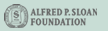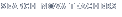Back to Teachers HomeHunting the Hidden DimensionClassroom ActivityActivity Summary
Students investigate fractals, learn how fractals can be used to determine the length of a coastline, and explore the expression of mathematical principles in art.

Learning Objectives
Students will be able to:

• state some of the characteristics of fractals.

• describe how fractals can be used to determine the length of coastlines.

• recognize mathematical principles in art and apply them to an artistic endeavor.

Suggested Time
Three to four class periods if all parts are completed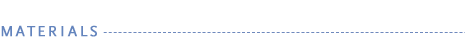Multimedia Resources

Part A

Part A

• several magnifying glasses
• pencil or pen

Part B

Part C

• art supplies such as paper, paints, and sculpting clay for each student
• books featuring a variety of artwork

Background

The term fractal was coined by French mathematician Benoît Mandelbrot in 1975. He based the term on the Latin word fractus, which means "broken." A fractal is a geometric figure with some special properties—it is irregular, fractured, or fragmented in appearance, and it is self-similar; that is, the figure looks much the same no matter how far away or how close up it is viewed. In addition, unlike most geometric shapes, fractals have infinite areas and perimeters.

Fractals can be found extensively in nature: clouds, trees, coastlines, and mountains can all be described as fractals. Because of this, fractal geometry has many practical applications. Geologists can model the meandering paths of rivers and the rock formations of mountains. Botanists can model the branching patterns of trees and shrubs. Astronomers can model the distribution of mass in the universe. Physiologists can model the human circulatory system. Physicists and engineers can model turbulence in fluids.

Fractal images are constructed by the iteration of a mathematical function, by repeatedly substituting certain geometric shapes with other shapes, or by repeatedly applying geometric transformations, such as rotation or reflection, to points. The results of the functions are then plotted on a graph, and the points are colored using a formula. (Mandelbrot's famous fractal set is constructed by the recursive iteration of the formula z = z2 + c, where z and c are complex numbers, and c is a constant.) Because of the complexity of these calculations, and the number of iterations required, fractals are usually generated on a computer.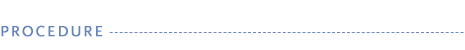Part A: A Closer Look at Fractals

Before the Lesson

1. Gather examples of fractals and non-fractals to bring to class. (Examples of fractals include a head of broccoli or cauliflower, a stem of a fern, or an image of snowflakes, blood vessels, the surface of the moon, or The Great Wave Off Kanagawa created by Katsushika Hokusai; non-fractals include anything with a traditional geometric shape, such as a ball, a square box, or a triangular prism.)

2. Obtain images of fractals to display for the class. Some images can be found at Fractal Art Main Gallery Web site.

3. Students will be viewing a video clip of Benoît Mandelbrot. Bookmark the video clip at the Peoples Archive: Benoît Mandelbrot Web site.

4. You can use the fractal imaging software as a teacher-led demonstration or have students work in teams with the application. Bookmark the Studying Mandelbrot Fractals Web site on your computer or on students' computers. The Java applet allows users to zoom in and out of a Mandelbrot fractal image. Instructions for using the online viewer are included on the Web page. Do all the steps of the interactive activity prior to using it with students.

The Lesson

1. Place the fractal and non-fractal objects together in two groups at the front of the class. Ask students to describe characteristics of each group. (Common characteristics of fractals include that they are irregularly shaped and are not simple to measure; non-fractals, on the other hand, follow standard geometric patterns and are fairly simple to measure.)

2. Show the class images of fractals. Which of the object groups that are in the front of the classroom do the images more closely resemble? Ask if anyone knows what these types of images are called. If no one suggests the term fractal, introduce the term to students and explain to them that the word was coined by the French mathematician Benoît Mandelbrot, who developed the concept of fractals in 1975.

3. Pass the images around and have students examine them more closely. Encourage students to use a magnifying glass to study different parts of the object. Ask what is noticeable about the magnified parts when they are compared to the whole fractal. (Fractals exhibit self-similarity, i.e., the same type of structures appear at any scale—small or large—at which the fractal is viewed.)

4. Tell students they will be investigating fractals to try to gain a better understanding of what they are. Have students visit the Peoples Archive: Benoît Mandelbrot Web site to view a video (3m 13s) of Benoît Mandelbrot describing fractals. Click on the Video menu "Medium" button. Have students take notes on characteristics of fractals that Mandelbrot mentions. (The key idea contained in this video is the concept that fractals are made of parts, and that each part is like the whole except smaller.) If students have difficulty understanding Mandelbrot in the video, they can read a transcript when the clip is over by clicking the "Read" button from the Transcript menu.

5. Next demonstrate or show students how to use the Studying Mandelbrot Fractals Java Interactive. Organize students into teams and have them work together to study the fractals. Students can use the "Col+" and "Col-" buttons to change the image's colors. Then use the mouse to draw a rectangle on the image and click "Go!" to enlarge the image. Have students zoom in on the fractal around its edges. Repeat using different parts of the fractal. What do students notice when the zoom in and out of the images? (Students should notice that the edges are jagged or irregular and that as they zoom in the images they see are similar to or the same as the original image.)

6. When students have finished viewing different fractal sets, hold a class discussion about their findings. Work as a class to come up with a definition for fractal. (A fractal is an irregular geometric object in which the parts are identical or similar to the whole when viewed at different scales, a concept known as self similarity.)

7. As an extension, have students collect or take pictures of fractals that they see in everyday life and share them with the class.

Part B: Jagged Edges of the Land

Before the Lesson

1. Download or copy a map or a high-resolution image of a local coastline or river (you can use Google Earth to find and zoom in to such a location). Coastlines that are jagged, rather than smooth, work better for this lesson. Examples are those found at Cape Cod, Massachusetts; Lake Superior, Michigan; and much of Alaska.

2. Gather all Part B materials each team will need.

The Lesson

1. Explain to students that Mandelbrot began his paper on fractal geometry by considering the question: "How long is the coast of Britain?" Tell students that in this activity they will be investigating the length of a local coastline.

2. Group the class into teams and distribute copies of the map, the Coastline Measurements student handout, and other materials.

3. Tell students that they will measure the coastline three times using the calipers or compass. Have teams select a unit of measurement, such as 2 centimeters, for their first test, and record that unit in the "Size of Step" column in their student handout table. (Note: Other tests will consist of using a measure that is half the length of the preceding test, so it is helpful to start with a measure that is easily divided by 2.) Have teams set the calipers or compass so that the distance between the points is equal to the unit of measure each team selects.

4. Demonstrate how to measure the coastline using the calipers or compass. Place one end of the tool at a beginning point on the map and then swing the tool so that the second end rests on the coastline near the starting point. Then leaving the second end in place, swing the first end to the next nearest point on the coast. Tell students that each "swing" represents one step along the coastline.

5. Have students use the calipers or compass to determine the actual number of steps that are needed. They should record their findings in the second column on the worksheet.

6. Have students calculate the length of the coastline by multiplying the total number of steps by the distance covered in each step and then record the result in the third column.

7. Next, have students repeat the exercise (Test 2), this time using a unit of measure that is half the original unit. When students have completed the second test, have them do a final test (Test 3) in which the unit of measure is half of the second unit.

8. After students have completed the activity, discuss their results. What happens to the total length of the coastline for different step sizes? (The coastline length becomes longer as the step size decreases.) What do you think the final length of the coastline would be if the step size kept decreasing? (The coastline would become infinitely long.) How is measuring the length of a coastline different from measuring the circumference of a circle or the distance around a square? (The measured length of a coastline depends on the length of the measuring tool you use.) Why is it important to measure coastlines? (Having accurate coastline measurements helps researchers determine whether and how coastlines are changing. That, in turn, can help scientists determine what forces are contributing to those changes.)

9. Point out that coastlines are considered natural fractals. Ask students to imagine applying the methods and results of this activity to finding the length of the edges of constructed fractals, such as the Koch Snowflake. What do they predict would happen they were to try to measure the edge of a fractal? (The length of the edge would depend on the length of the ruler they used to measure the edge. The smaller the length of measurement, the longer the perimeter of the fractal.)

Part C: The Art of Math

Before the Lesson

1. Gather all Part C materials each team will need.

The Lesson

1. Begin by asking students to consider what, if any, relationship exists between art and mathematics. Ask students to give examples of mathematical principles and/or concepts that are used in art. (Responses will vary, but may include such examples as perspective, geometry, tessellations, patterns, topology, and fractals.) Write their responses on the board. Next, ask students if they can think of any artists who use mathematical principles or concepts in their work. Write any suggestions students might have on the board.

2. Have students choose a piece of artwork they like that they think exhibits mathematical principles. Have them find the artwork in books or online, print out their chosen artwork, and write a paragraph describing the principles involved.

3. Tell students they will now be applying the mathematical principles they found in the artwork they selected to a piece of their own. Allow students to work in their medium of choice, such as a sketch, painting, sculpture, or computer graphics rendering.

4. After students have completed their creations, invite them to share their finished works with the class. Ask students in the "audience" to identify which mathematical principles are exhibited in each piece; then have the student who is presenting the work confirm whether those were the principles he or she set out to include.

5. When all students have presented, discuss the different types of art represented. Are all the pieces strictly geometrical or abstract? If not, what are some of the other styles of art represented? What types of media were used? How did the artists incorporate mathematics into their pieces? What, if anything, do mathematics and art have in common?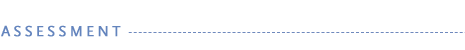Use the following rubric to assess students' work.

 Excellent Satisfactory Needs Improvement Part A: A Closer Look at Fractals Students are active participants in class discussions. They can identify some of the defining characteristics of fractals and can express those characteristics. They can use the software independently and accurately. Students participate in class discussions, and can identify some of the defining characteristics of fractals, but may not be able to easily express what those characteristics are. They may need assistance using the software. Students do not participate in class discussions, and may have difficulty recognizing some of the defining characteristics of fractals. They have difficulties using the software. Part B: Jagged Edges of the Land Students work cooperatively and record their measurements and calculations accurately on the worksheet. They are able to describe their results and draw conclusions. Students work cooperatively, however there may be minor errors or missing details in their work. They demonstrate a general understanding, but may need help drawing conclusions about their results. Students do not work in a cooperative manner. There are notable gaps or errors in their work. They have difficulties describing their results and/or drawing conclusions. Part C: The Art of Math Students are able to identify math concepts in a piece of chosen art and then apply those concepts to artwork they develop. Students cannot identify all the math concepts in their chosen artwork and/or can only apply one of those concepts to their own art. Students make little effort to find math in the art they choose and cannot transfer that knowledge to their own piece of artwork.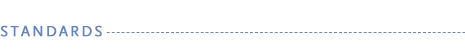The "Hunting the Hidden Dimensions" activity aligns with the following Principles and Standards for School Mathematics.

Algebra
Geometry
Number and Operation

Algebra
Measurement

Classroom Activity Author

Margy Kuntz has written and edited educational materials for more than 24 years. She has authored numerous educational supplements, basal text materials, and trade books on science, math, and computers.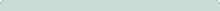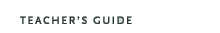Hunting the Hidden Dimension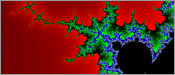Original broadcast:October 28, 2008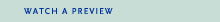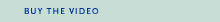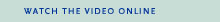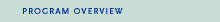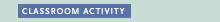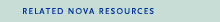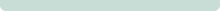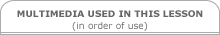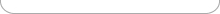Funding for NOVA is provided by ExxonMobil, David H. Koch, the Howard Hughes Medical Institute, the Corporation for Public Broadcasting, and public television viewers.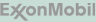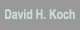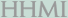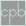Major funding for "Hunting the Hidden Dimension" is provided by the Alfred P. Sloan Foundation.